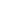科研动态最新资讯

• 天元讲堂（12.9）：Multiple expansions of real numbers with digits set $\{0,1,q\}$
• 浏览量：10 发布人： 发布时间：2018-12-06
• 报告人： 李文侠教授 （华东师范大学）

题目：Multiple expansions of real numbers  with  digits set $\{0,1,q\}$

报告时间： 2018年12月9日（周日）下午 3：30-4：30

报告地点： 数学二楼报告厅

摘要：For $q>1$  we consider expansions in base $q$ over the alphabet $\{0,1,q\}$. Let ${\mathcal U}_q$ be the set of $x$ which have a unique $q$-expansions. For   $k=2, 3,\cdots,\aleph_0$ let ${\mathcal B}_k$ be the set of bases $q$ for which there exists $x$ having precisely $k$ different  $q$-expansions, and  for $q\in {\mathcal B}_k$ let ${\mathcal U}_q^{(k)}$ be the set of all such  $x$'s which have exactly $k$ different $q$-expansions. In this paper we show that

${\mathcal B}_{\aleph_0}=[2,\infty)\quad\textrm{and}\quad {\mathcal B}_k=(q_c,\infty)\quad \textrm{for any}\quad k\ge 2,$

where

$q_c\approx 2.32472$ is the appropriate root of

$x^3-3x^2+2x-1=0$.

Moreover,  we show that for any positive integer $k\ge 2$ and any  $q\in{\mathcal B}_{k}$ the Hausdorff dimensions of ${\mathcal U}_q^{(k)}$  and ${\mathcal U}_q$ are   the same, i.e.,

$$\dim_H{\mathcal U}_q^{(k)}=\dim_H{\mathcal U}_q\quad\textrm{for any}\quad k\ge 2.$$

Finally, we conclude that the set of $x$ having a continuum of $q$-expansions has full Hausdorff dimension.